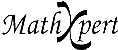# Help With Math: Purveyors of MathXpert

-->## MathXpert: software to help you learn mathematics.### How does MathXpert solve problems? Does it use artificial intelligence?

 MathXpert is based on the following view of mathematics: there are operations that we use to transform mathematical expressions. For example, the operation "collect like terms" can change 3x + 2x to 5x. We solve a problem by applying one operation at a time until our initial problem is transformed to a desired form that we consider the answer. That means there are two parts to learning mathematics: You must learn what the individual operations are and how they work, and you must learn how to decide what operation to apply next. Usually in courses and textbooks, the focus is on the individual operations, but knowing what to do when is often a source of difficulty for students. MathXpert's problem-solving mechanism has two corresponding parts: First, there is computer code for carrying out each of some 1600 mathematical operations. Second, there is computer code for managing MathXpert's "auto mode" in which it can determine which operation to apply next, and where to apply it. The computer implementation of the mathematical operations, while time-consuming and demanding, is not artificial intelligence. The rules for what operation to apply when is indeed a classical application of artificial intelligence technology. These are "rules about rules", or "meta-rules". So far as the author of MathXpert knows, they have never before been explicitly formulated--not even in answer-only mathematical software such as Mathematica or Maple, since if all you need is the answer, the order of operations is often not so critical. You can now benefit from this technology: in effect you have an "expert system" in mathematics at your elbow to guide you. In MathXpert, the ability of this expert system to solve problems can be tapped in several ways that may be more helpful to you or your students than simply presenting a complete solution at one click of the mouse. The internally-generated solution can be used to generate hints, or to show the student what step would be good to take next. The feeling of having an expert at one's elbow reduces frustration and increases confidence, as well as being of direct pedagogical value.